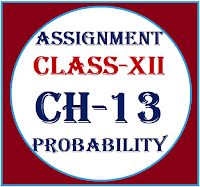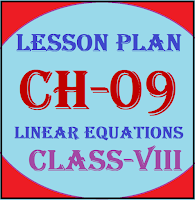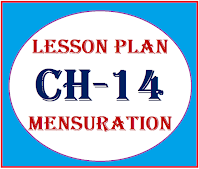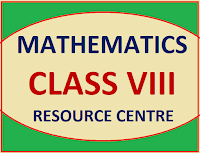## Posts

### Common Errors in Secondary Mathematics

Common Errors Committed  by the  Students  in Secondary Mathematics   Errors  that students often make in doing secondary mathematics  during their practice and during the examinations  and their remedial measures are well explained here stp by step.  Some Common Errors in Mathematics

### Math Assignment Class XII | ProbabilityMathematics Assignment on  Probability Class XII

### Lesson Plan Math Class 8 | Linear Equations In One Variable CH-09E- LESSON PLAN MATHEMATICS  CLASS- 8, CH-09 Lesson Plan for CBSE mathematics class 8 Linear Equations in one variable, Step by step teaching strategy for mathematics teachers. Perfect lesson plan which makes the teaching learning process perfect LESSON PLAN MATHEMATICS LINEAR EQUATIONS IN ONE VARIABLE RMB DAV CENTENARY PUBLIC SCHOOL NAWANSHAHR NAME OF THE TEACHER DINESH KUMAR CLASS VIII CHAPTER 09 SUBJECT MATHEMATICS TOPIC LINEAR EQUATIONS IN ONE VARIABLE  DURATION : 16 Class Meetings PRE-REQUISTIC KNOWLEDGE Basic Arithmetic: Students should have a strong grasp of basic arithmetic operations, including addition, subtraction, multiplication, and division. These operations are the building blocks of solving linear equations.

### Lesson Plan Math Class 8 | Mensuration CH-14E- LESSON PLAN SUBJECT MATHEMATICS CLASS- 8, CH-14 Lesson Plan for CBSE mathematics class 8 Mensuration, Step by step teaching strategy for mathematics teachers. Perfect lesson plan which makes the teaching learning process perfect E-LESSON PLAN MATHEMATICS CLASS-VIII CHAPTER - 14 MENSURATION RMB DAV CENTENARY PUBLIC SCHOOL NAWANSHAHR NAME OF THE TEACHER DINESH KUMAR CLASS VIII CHAPTER 14 SUBJECT MATHEMATICS TOPIC MENSURATION  DURATION : 15 Class Meetings PREREQUISITE KNOWLEDGE FOR THIS LESSON PLAN BASIC GEOMETRY: Students should have a good understanding of basic geometric shapes like rectangles, squares, triangles, and circles. PERIMETER AND AREA CONCEPTS: Prior knowledge of the concepts of perimeter and area is essential. Students should understand that the perimeter is the total distance around a shape, while area represents the space enclosed

### Maths Class VIII Formulas, Assignments & Lesson PlanMATHEMATICS CLASS VIII  FORMULAS, ASSIGNMENTS & LESSON PLAN   Class-VIII (MATHEMATICS) Chapter 1 : Square & Square Root